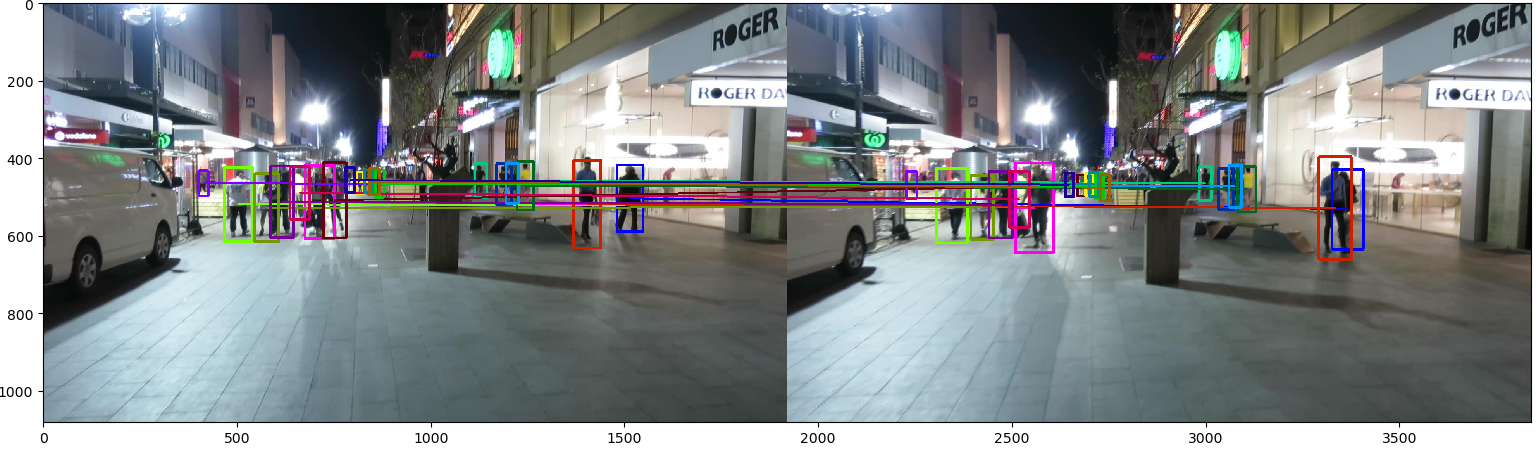## 前言

Kalman滤波器是多目标跟踪任务中一个经典的运动模型，本次主要以代码实践进行讲解。下文中所有的代码都会开源在https://github.com/nightmaredimple/libmot。

## 1Kalman Filter

$$\begin{array}{l}x = Fx + Bu\\P = \alpha FxF^T + Q\end{array}$$

$$\left\{ \begin{array}{l} K = PH^T/\left( {HPH^T + R} \right)\\ x = Hx + K\left( {z - Hx} \right)\\ P = (1 - KH)P \end{array} \right.$$

$$P = \left( {1 - KH} \right)P{\left( {1 - KH} \right)^T} + KR{K^T}$$

## 3Example

Step1 我们先初始化参数：

Step2 读取detection和groundtruth文件，筛选出满足行人类别和检测置信度阈值的目标：

Step3 对每个目标新建一个Kalman滤波器，逐一进行预测、更新、数据关联。其中如果数据关联失败的话，对于匹配失败的跟踪轨迹，在一定时间内，我们依旧允许其预测。

Step4 我们将轨迹中有效长度较短的轨迹视为无效轨迹：

DetectionMOTA↑MOTP↑IDF1↑ID Sw.↓
SDP0.6750.2030.518201## 参考资源

WOJKE N, BEWLEY A, PAULUS D. Simple online and realtime tracking with a deep association metric[C]. in: 2017 IEEE international conference on image processing (ICIP). IEEE, 2017. 3645-3649.

-------------本文结束感谢您的阅读-------------